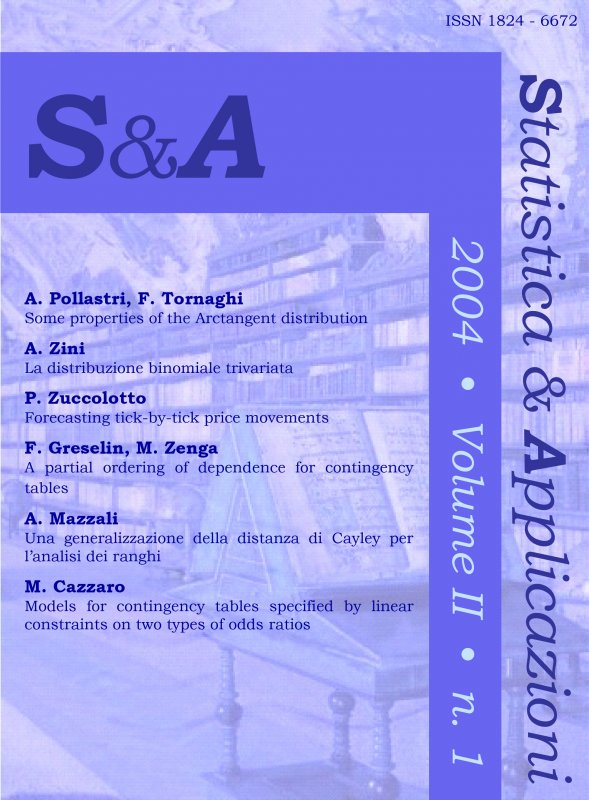Your browser does not support JavaScript!

# STATISTICA & APPLICAZIONI - 2004 - 1

digitalDigital issue
 journal STATISTICA & APPLICAZIONI issue 1 - 2004 title STATISTICA & APPLICAZIONI - 2004 - 1 publisher Vita e Pensiero formatDigital issue |Pdf language English Write a comment for this product

### Ebook format Pdf readable on these devices:

• Computer
with Software:
• Android
• iPhone/Ipad
with App:
• E-Book Reader
• Ibs Tolino
• Kobo
Primo fascicolo del 2004

#### CONTENTS

##### Some properties of the Arctangent Distribution
by Angiola Pollastri, Franco Tornaghi pages: 16
Abstract
In the present paper some characteristics of the Arctangent Distribution are analysed. Arctangent was utilised in order to obtain simultaneous confidence intervals of the probabilities of the multinomial distribution. In particular, the asimmetry and the relations with the Folded Standard Normal and the Folded Skew Normal are studied. Then the distribution of the maximum and of the minimum of the absolute value of the component of a Bivariate Correlated Normal r.v are studied and used to improve confidence region and corresponding tests of hypotheses for the two means of a Bivariate Correlated Normal.

Keywords: Asymmetry, Folded Standard Normal, Skew–Normal distribution, Bivariate Correlated Normal.
##### La distribuzione binomiale trivariata
pages: 18
Abstract
Several categorical data analyses, consider dichotomous characters imposing either statistical independence or multinomial dependence, ignoring which the natural statistical models of dependence are.
The binomial bivariate distribution, defined by Zenga (1968), is the natural structure of the number of successes of two characters in n independent extractions. This work generalises it to three characters: the binomial trivariate distribution, which exhibits univariate margins binomial and bivariate margins binomial bivariate, is defined and studied. Marginal and conditional distributions of the binomial trivariate distribution will be deduced, and, from them, formulae for expectations and variances will be derived.

Keywords: Distribuzione Binomiale Bivariata, Funzione Generatrice dei Momenti, Distribuzione Multinomiale.
##### Forecasting tick-by-tick price movements
by Paola Zuccolotto pages: 16
Abstract
This paper proposes a new technique aimed to forecasting the price movements in the context of ultra-high frequency data, based on the theory of ACD models. The approach to the problem consists in the use of the conditional means of different financial durations to calculate the probabilities of price movements, as an alternative to the traditional custom in specifying a parametric model for the dynamics of price. An application is presented on a stock of Italian financial market.

Keywords: Ultra-high frequency data, durations, ACD models, price movements.
##### A partial ordering of dependence for contingency tables
by Francesca Greselin, Michele Zenga pages: 19
Abstract
For two-way contingency tables, whose row and column variables have nominal categories, we introduce a new partial order according to the strength of dependence within the class of all contingency tables with given margins. The order holds among tables which share the same configuration of signs in the deviation from independence (contingencies) and takes into account the absolute value of these contingencies. Our order, which seems to possess a more direct and intuitive interpretation relative to similar ones available in the literature, is characterized by a sequence of frequency transfers over a closed path, which involve an even number of cells and leave the marginal distributions unchanged. We derive necessary and sufficient conditions for maximal (minimal) tables, according to our notion of dependence. Furthermore a simple algorithm is provided to check these conditions. Finally, an associated measure of dependence is briefly mentioned.

Keywords:Dependence ordering, stochastic ordering, contingency tables, measures of dependence, bivariate distributions with given margins.
##### Una generalizzazione della distanza di Cayley per l’analisi dei ranghi
by Aride Mazzali pages: 16
Abstract
In this paper we consider the Cayley’s distance for rank data and a generalization that we are going to introduce. The Cayley’s distance is drawn by the number of the inversions in the permutation function derived composing ranking and ordering functions, while the generalized distance comes from a monotonic non decreasing transformation of the previous one. The generalized distance is then used to define the center and the spread of a data set. The important concepts of total and relative disorder are also introduced as measures that seem useful to cluster and to analyze the respondents’ behaviour in either full and partial ranking. An application to the Diaconis’ data-set is shown.

Keywords: Ranking, Ordering, Inversions, Cayley’s distance, Disorder.
##### Models for contingency tables specified by linear constraints on two types of odds ratios
by Manuela Cazzaro pages: 12
Abstract
A class of models for multi-way contingency tables obtained from a log-linear model by imposing non linear constraints on the interaction parameters are presented. In particular the constraints will regard measures of bivariate association between a pair of categorical variables based on the local-continuation and continuation-local odds ratios.
The contribution of this work, according to the author's knowledge, is the simultaneous constraining of more than one type of odds ratio and the approach to manage the row and the column effect of the dependence relationship between categorical variables.

Keywords: Log-linear Models, Logit Models, Generalised Odds Ratios, Constrained Maximum Likelihood.